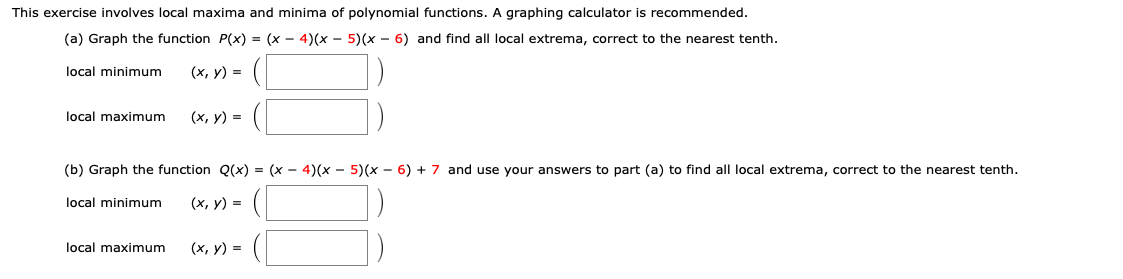# This exercise involves local maxima and minima of polynomial functions. A graphing calculator is recommended. (a) Graph the function P(x) = (x - 4)(x - 5) (x - 6) and find all local extrema, correct to the nearest tenth (х, у) %3D local minimum local maximum (х, у) 3D (b) Graph the function Q(x) = (x - 4)(x - 5)(x - 6) + 7 and use your answers to part (a) to find all local extrema, correct to the nearest tenth. (х, у) %3D local minimum local maximum (х, у) %3

Questionhelp_outlineImage TranscriptioncloseThis exercise involves local maxima and minima of polynomial functions. A graphing calculator is recommended. (a) Graph the function P(x) = (x - 4)(x - 5) (x - 6) and find all local extrema, correct to the nearest tenth (х, у) %3D local minimum local maximum (х, у) 3D (b) Graph the function Q(x) = (x - 4)(x - 5)(x - 6) + 7 and use your answers to part (a) to find all local extrema, correct to the nearest tenth. (х, у) %3D local minimum local maximum (х, у) %3 fullscreen

## Expert Answer

### Want to see this answer and more?

Experts are waiting 24/7 to provide step-by-step solutions in as fast as 30 minutes!*

*Response times vary by subject and question complexity. Median response time is 34 minutes and may be longer for new subjects.
Tagged in
MathCalculus

### Derivative© 2021 bartleby. All Rights Reserved.